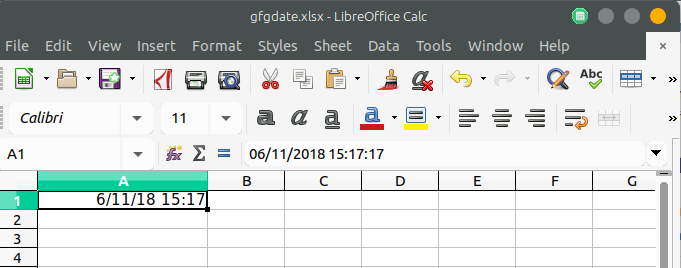# PHP | Spreadsheet | Setting a date and/or time value in a cell

• Difficulty Level : Easy
• Last Updated : 19 Nov, 2018

In PHP Spreadsheet, the date or time values are stored in an excel sheet in the form of timestamp which is a floating point value. So in order to store date/time in a human-readable format, need to calculate the correct excel timestamp and finally set a number format mask.

Example:

 `getActiveSheet(); ``  ` `// Set the number format mask so that the excel timestamp ``// will be displayed as a human-readable date/time``\$spreadsheet``->getActiveSheet()->getStyle(``'A1'``)``    ``->getNumberFormat()``    ``->setFormatCode(``    ``\PhpOffice\PhpSpreadsheet\Style\NumberFormat::FORMAT_DATE_DATETIME``    ``);``  ` `// Get current date and timestamp``// Convert to an Excel date/time``\$dateTime` `= time(); ``\$excelDateValue` `= \PhpOffice\PhpSpreadsheet\Shared\``Date``::PHPToExcel(``                  ``\$dateTime` `); ``  ` `// Set cell A1 with the Formatted date/time value``\$sheet``->setCellValue(``'A1'``,``\$excelDateValue``);``  ` `// Write an .xlsx file ``\$writer` `= ``new` `Xlsx(``\$spreadsheet``);`` ` `// Save .xlsx file to the current directory``\$writer``->save(``'gfgdate.xlsx'``);``?>`

Output:My Personal Notes arrow_drop_up